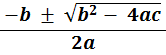### Answer − Solving quadratics of the form ax2 + bx + c = 0 using the quadratic formula

#### 1.   Solve 2x2 + 10x + 8 = 0

 x =Verify by putting these two values of x into 2x2 + 10x + 8

#### 2.   Find the values of x where 3x2 + 21x + 18 = 0

 x =Verify by putting these two values of x into 3x2 + 21x + 18

#### 3.   Solve 4x2 + 20x − 24 = 0

 x =Verify by putting these two values of x into 4x2 + 20x − 24

#### 4.   Find the values of x where 2x2 + 9x − 56 = 0

 x =Verify by putting these two values of x into 2x2 + 9x − 56

#### 5.   Solve 5x2 − 20x − 60 = 0

 x =Verify by putting these two values of x into 5x2 − 20x − 60

#### 6.   Find the values of x where 3x2 − 21x + 36 = 0

 x =Verify by putting these two values of x into 3x2 − 21x + 36

#### 7.   Solve 3x2 − 40x + 48 = 0

 x =Verify by putting these two values of x into 3x2 − 40x + 48back to: